# Oscillator Circuits

## Discrete Semiconductor Devices and Circuits

• #### Question 1

 Don’t just sit there! Build something!!

Learning to mathematically analyze circuits requires much study and practice. Typically, students practice by working through lots of sample problems and checking their answers against those provided by the textbook or the instructor. While this is good, there is a much better way.

You will learn much more by actually building and analyzing real circuits, letting your test equipment provide the “answers” instead of a book or another person. For successful circuit-building exercises, follow these steps:

1. Carefully measure and record all component values prior to circuit construction, choosing resistor values high enough to make damage to any active components unlikely.
2. Draw the schematic diagram for the circuit to be analyzed.
3. Carefully build this circuit on a breadboard or other convenient medium.
4. Check the accuracy of the circuit’s construction, following each wire to each connection point, and verifying these elements one-by-one on the diagram.
5. Mathematically analyze the circuit, solving for all voltage and current values.
6. Carefully measure all voltages and currents, to verify the accuracy of your analysis.
7. If there are any substantial errors (greater than a few percent), carefully check your circuit’s construction against the diagram, then carefully re-calculate the values and re-measure.

When students are first learning about semiconductor devices, and are most likely to damage them by making improper connections in their circuits, I recommend they experiment with large, high-wattage components (1N4001 rectifying diodes, TO-220 or TO-3 case power transistors, etc.), and using dry-cell battery power sources rather than a benchtop power supply. This decreases the likelihood of component damage.

As usual, avoid very high and very low resistor values, to avoid measurement errors caused by meter “loading” (on the high end) and to avoid transistor burnout (on the low end). I recommend resistors between 1 kΩ and 100 kΩ.

One way you can save time and reduce the possibility of error is to begin with a very simple circuit and incrementally add components to increase its complexity after each analysis, rather than building a whole new circuit for each practice problem. Another time-saving technique is to re-use the same components in a variety of different circuit configurations. This way, you won’t have to measure any component’s value more than once.

• #### Question 2

The circuit shown here is called a relaxation oscillator. It works on the principles of capacitor charging over time (an RC circuit), and of the hysteresis of a gas-discharge bulb: the fact that the voltage required to initiate conduction through the bulb is significantly greater than the voltage below which the bulb ceases to conduct current.

In this circuit, the neon bulb ionizes at a voltage of 70 volts, and stops conducting when the voltage falls below 30 volts:Graph the capacitor’s voltage over time as this circuit is energized by the DC source. Note on your graph at what times the neon bulb is lit:

• #### Question 3

Replace the fixed-value resistor with a potentiometer to adjust the blinking rate of the neon lamp, in this relaxation oscillator circuit. Connect the potentiometer in such a way that clockwise rotation of the knob makes the lamp blink faster:• #### Question 4

Define what an oscillator circuit is, using your own words. Give a few examples of oscillators at work in common devices and systems.

• #### Question 5

This relaxation oscillator circuit uses a resistor-capacitor combination (R1 - C1) to establish the time delay between output pulses:The voltage measured between TP1 and ground looks like this on the oscilloscope display: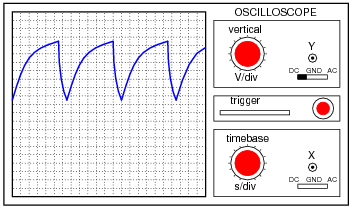A slightly different version of this circuit adds a JFET to the capacitor’s charge current path:Now, the voltage at TP1 looks like this: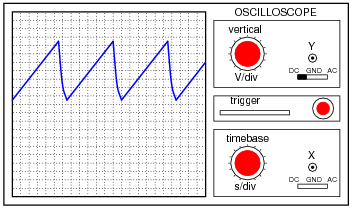What function does the JFET perform in this circuit, based on your analysis of the new TP1 signal waveform? The straight-line charging voltage pattern shown on the second oscilloscope display indicates what the JFET is doing in this circuit.

Hint: you don’t need to know anything about the function of the unijunction transistor (at the circuit’s output) other than it acts as an on/off switch to periodically discharge the capacitor when the TP1 voltage reaches a certain threshold level.

Challenge question: write a formula predicting the slope of the ramping voltage waveform measured at TP1.

• #### Question 6

This circuit shown here is for a timing light: a device that uses a pulsed strobe lamp to “freeze” the motion of a rotating object.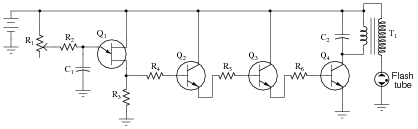Which component(s) in this circuit form the oscillator section? What type of oscillator is used in this circuit? Which component values have a direct influence on the frequency of the flash tube’s output?

• #### Question 7

Explain the principle of operation in this astable multivibrator circuit: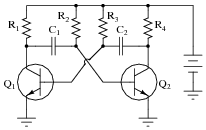Also, identify where you would connect to this circuit to obtain an output signal. What type of signal would it be (sine wave, square wave, ramp or triangle wave, etc.)?

• #### Question 8

This astable multivibrator circuit will oscillate with a 50% duty cycle if the components are symmetrically sized:Determine which component(s) would have to be re-sized to produce a duty cycle other than 50%.

• #### Question 9

If you have ever used a public address (“PA”) amplifier, where sounds detected by a microphone are amplified and reproduced by speakers, you know how these systems can create “screeching” or “howling” sounds if the microphone is held too close to one of the speakers.

The noise created by a system like this is an example of oscillation: where the amplifier circuit spontaneously outputs an AC voltage, with no external source of AC signal to “drive” it. Explain what necessary condition(s) allow an amplifier to act as an oscillator, using a “howling” PA system as the example. In other words, what exactly is going on in this scenario, that makes an amplifier generate its own AC output signal?

• #### Question 10

How many degrees of phase shift must the feedback circuit (the box in this schematic) introduce to the signal in order for this common-emitter amplifier circuit to oscillate?We know that oscillator circuits require “regenerative” feedback in order to continuously sustain oscillation. Explain how the correct amount of phase shift is always provided in the feedback circuit to ensure that the nature of the feedback is always regenerative, not degenerative. In other words, explain why it is not possible to incorrectly choose feedback network component values and thus fail to achieve the proper amount of phase shift.

• #### Question 11

How many degrees of phase shift must the feedback circuit (the box in this schematic) introduce to the signal in order for this two-stage common-emitter amplifier circuit to oscillate?Why is this amount of phase shift different from that of a single-transistor oscillator?

• #### Question 12

Explain what the Barkhausen criterion is for an oscillator circuit. How will the oscillator circuit’s performance be affected if the Barkhausen criterion falls below 1, or goes much above 1?

• #### Question 13

One way to achieve the phase shift necessary for regenerative feedback in an oscillator circuit is to use multiple RC phase-shifting networks:What must the voltage gain be for the common-emitter amplifier if the total voltage attenuation for the three phase-shifting RC networks is -29.25 dB?

• #### Question 14

RC phase-shift oscillator circuits may be constructed with different numbers of RC sections. Shown here are schematic diagrams for three- and four-section RC oscillators:What difference will the number of sections in the oscillator circuit make? Be as specific as you can in your answer.

• #### Question 15

Calculate the output voltages of this Wien bridge circuit, if the input voltage is 10 volts RMS at a frequency of 159.155 Hz: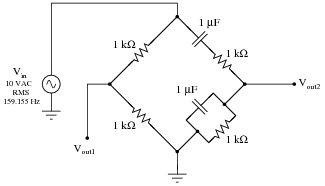• #### Question 16

In this Wien bridge circuit (with equal-value components all around), both output voltages will have the same phase angle only at one frequency:At this same frequency, Vout2 will be exactly one-third the amplitude of Vin. Write an equation in terms of R and C to solve for this frequency.

• #### Question 17

The circuit shown here is a Wien-bridge oscillator:If one side of the Wien bridge is made from a potentiometer instead of two fixed-value resistors, this adjustment will affect both the amplitude and the distortion of the oscillator’s output signal:Explain why this adjustment has the effect that it does. What, exactly, does moving the potentiometer do to the circuit to alter the output signal? Also, calculate the operating frequency of this oscillator circuit, and explain how you would make that frequency adjustable as well.

• #### Question 18

Identify the type of oscillator circuit shown in this schematic diagram, and explain the purpose of the tank circuit (L1 and C1):Also, write the equation describing the operating frequency of this type of oscillator circuit.

• #### Question 19

Identify the type of oscillator circuit shown in this schematic diagram, and explain the purpose of the tank circuit (L1, C1, and C2):Also, write the equation describing the operating frequency of this type of oscillator circuit.

• #### Question 20

Describe the purpose and operation of a crystal in an oscillator circuit. What physical principle does the crystal exploit, and what other components could be substituted in place of a crystal in an oscillator circuit?

• #### Question 21

Identify the type of oscillator circuit shown in this schematic diagram, and explain the purpose of the crystal:Challenge question: this type of oscillator circuit is usually limited to lower power outputs than either Hartley or Colpitts designs. Explain why.

• #### Question 22

A clever way to produce sine waves is to pass the output of a square-wave oscillator through a low-pass filter circuit: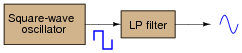Explain how this principle works, based on your knowledge of Fourier’s theorem.

• #### Question 23

Predict how the operation of this relaxation oscillator circuit will be affected as a result of the following faults. Consider each fault independently (i.e. one at a time, no multiple faults):Capacitor C1 fails open:
Capacitor C1 fails shorted:
Resistor R1 fails open:
Solder bridge (short) past resistor R1:

For each of these conditions, explain why the resulting effects will occur.

• #### Question 24

Predict how the operation of this strobe light circuit will be affected as a result of the following faults. Consider each fault independently (i.e. one at a time, no multiple faults):Capacitor C1 fails open:
Capacitor C1 fails shorted:
Resistor R2 fails open:
Solder bridge (short) past resistor R2:
Resistor R4 fails open:
Transistor Q4 fails open (collector-to-emitter):
Capacitor C2 fails open:
Capacitor C2 fails shorted:

For each of these conditions, explain why the resulting effects will occur.

• #### Question 25

Predict how the operation of this sawtooth-wave oscillator circuit will be affected as a result of the following faults. Consider each fault independently (i.e. one at a time, no multiple faults):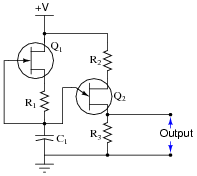Capacitor C1 fails shorted:
Resistor R1 fails open:
JFET fails shorted (drain-to-source):
Resistor R3 fails open:

For each of these conditions, explain why the resulting effects will occur.

• #### Question 26

Predict how the operation of this astable multivibrator circuit will be affected as a result of the following faults. Specifically, identify the final states of the transistors (on or off) resulting from each fault. Consider each fault independently (i.e. one at a time, no multiple faults):Capacitor C1 fails open:
Capacitor C2 fails open:
Resistor R1 fails open:
Resistor R2 fails open:
Resistor R3 fails open:
Resistor R4 fails open:

For each of these conditions, explain why the resulting effects will occur.

• #### Question 27

Predict how the operation of this astable multivibrator circuit will be affected as a result of the following faults. Specifically, identify the signals found at test points TP1, TP2, TP3, and Vout resulting from each fault. Consider each fault independently (i.e. one at a time, no multiple faults):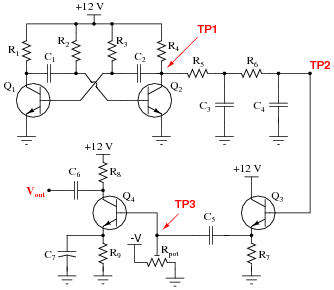Resistor R4 fails open:
Resistor R5 fails open:
Resistor R7 fails open:
Resistor R9 fails open:
Capacitor C7 fails shorted:
Capacitor C4 fails shorted:
Capacitor C5 fails open:
Transistor Q3 fails open (collector-to-emitter):

For each of these conditions, explain why the resulting effects will occur.

• #### Question 28

Identify some realistic component failures that would definitely prevent this oscillator circuit from oscillating:For each of the faults you propose, explain why the oscillations will cease.

• #### Question 29

Suppose some of the turns of wire (but not all) in the primary winding of the transformer were to fail shorted in this Armstrong oscillator circuit: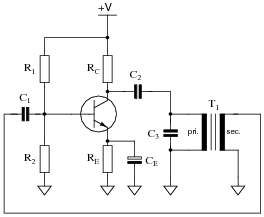How would this effective decreasing of the primary winding turns affect the operation of this circuit? What if it were the secondary winding of the transformer to suffer this fault instead of the primary?

• #### Question 30

A technician is given a transistor testing circuit to repair. This simple circuit is an audio-frequency oscillator, and has the following schematic diagram:After repairing a broken solder joint, the technician notices that the DPDT switch has lost its label. The purpose of this switch is to allow polarity to be reversed so as to test both PNP and NPN transistor types. However, the label showing which direction is for NPN and which direction is for PNP has fallen off. And, to make matters worse, the schematic diagram does not indicate which position is which.

Determine what the proper DPDT switch label should be for this transistor tester, and explain how you know it is correct. Note: you do not even have to understand how the oscillator circuit works to be able to determine the proper switch label. All you need to know is the proper voltage polarities for NPN and PNP transistor types.

• #### Question 31

This electric fence-charging circuit, which is designed to produce short, high-voltage pulses on its output, has failed. Now, it produces no output voltage at all:A technician does some troubleshooting and determines that the transistor is defective. She replaces the transistor, and the circuit begins to work again, its rhythmic output pulses indicated by the neon lamp.

But after producing only a few pulses, the circuit stops working. Puzzled, the technician troubleshoots it again and finds that the transistor has failed (again). Both the original and the replacement transistor were of the correct part number for this circuit, so the failure is not due to an incorrect component being used. Something is causing the transistor to fail prematurely. What do you suppose it is?

• #### Question 32

Spring- and weight-driven clock mechanisms always use a pendulum as an integral part of their workings. What function does a pendulum serve in a clock? What would a mechanical clock mechanism do if the pendulum were removed?

Describe what the electrical equivalent of a mechanical pendulum is, and what purpose it might serve in an oscillator circuit.

• #### Question 33

Two technicians are arguing over the function of a component in this oscillator circuit. Capacitor C1 has failed, and they are debating over the proper value of its replacement.One technician argues that the value of capacitor C1 helps set the oscillation frequency of the circuit, and that the value of the replacement capacitor therefore must be precisely matched to the value of the original. The other technician thinks its value is not critical at all, arguing that all it does is help to provide a stable DC power supply voltage. What do you think?

Also, describe the purpose of this circuit: what is it?

• #### Question 34

How many degrees of phase shift must the feedback circuit (the square box in this schematic) introduce to the signal in order for this inverting amplifier circuit to oscillate?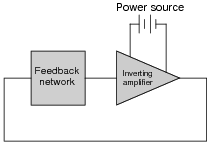• #### Question 35

How many degrees of phase shift must the feedback circuit (the square box in this schematic) introduce to the signal in order for this noninverting amplifier circuit to oscillate?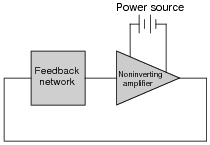• #### Question 36

Identify the type of oscillator circuit shown in this schematic diagram, and explain the purpose of the tank circuit (L1 and C1):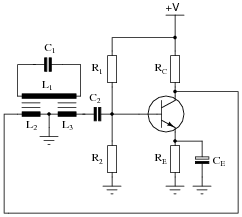Also, write the equation describing the operating frequency of this type of oscillator circuit.

• #### Question 37

Identify the type of oscillator circuit shown in this schematic diagram:Also, write the equation describing the operating frequency of this type of oscillator circuit.

• #### Question 38

Identify the type of oscillator circuit shown in this schematic diagram, and draw the transformer phasing dots in the right places to ensure regenerative feedback:Also, write the equation describing the operating frequency of this type of oscillator circuit.

• #### Question 39

Modify the schematic diagram for a Hartley oscillator to include a crystal. What advantage(s) does a crystal-controlled Hartley oscillator exhibit over a regular Hartley oscillator?

• #### Question 40

How does the quality factor (Q) of a typical quartz crystal compare to that of a regular LC tank circuit, and what does this indicate about the frequency stability of crystal-controlled oscillators?

• #### Question 41

Under certain conditions (especially with certain types of loads) it is possible for a simple one-transistor voltage amplifier circuit to oscillate: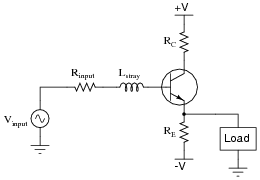Explain how this is possible. What parasitic effects could possibly turn an amplifier into an oscillator?

• #### Question 42

Calculate the operating frequency of this oscillator circuit:Explain why the operating frequency will not be the same if the transistor receives its feedback signal from the other side of the bridge, like this: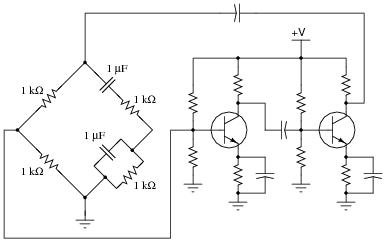• #### Question 43

This circuit generates quasi-sine waves at its output. It does so by first generating square waves, integrating those square waves (twice) with respect to time, then amplifying the double-integrated signal:Identify the sections of this circuit performing the following functions:

Square wave oscillator:
First integrator stage:
Second integrator stage:
Buffer stage (current amplification):
Final gain stage (voltage amplification):
• #### Question 44

Calculate the operating frequency of the following oscillator circuit, if C1 = 0.033 μF and L1 = 175 mH: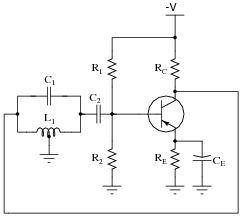• #### Question 45

Calculate the operating frequency of the following oscillator circuit, if C1 = 0.047 μF and L1 = 150 mH:• #### Question 46

Calculate the operating frequency of the following oscillator circuit, if C1 = 0.027 μF and L1 = 105 mH:• #### Question 47

Calculate the operating frequency of the following oscillator circuit, if C1 = 0.003 μF, C2 = 0.003 μF, and L1 = 50 mH:• #### Question 48

Calculate the operating frequency of the following oscillator circuit, if C1 = 0.005 μF, C2 = 0.005 μF, and L1 = 80 mH: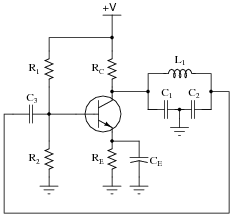• #### Question 49

Calculate the operating frequency of the following oscillator circuit, if C1 = 0.027 μF, C2 = 0.027 μF, and L1 = 220 mH: